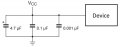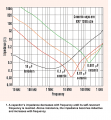# Bypass capacitor mechanism

#### k1ng 1337

Joined Sep 11, 2020
539
Greetings,

For some time I have been trying to understand the mechanism for bypass capacitors being used to eliminate power supply noise to galvanically connected devices. Each guide I have found describes what the the capacitor does and why it does but does not go into detail of how (the mathematics). Specifically, guides and datasheets say multiple bypass capacitors (of different values) placed in parallel will reject additional frequencies... how?

Consider the diagram (on a solderless breadboard):My theory is this: Each capacitor combined with the parasitic inductance (and resistance?) of nearby components creates a band stop filter (concerning the device) at the resonance frequency; using multiple capacitors in parallel creates an array of band stop filters whos frequency bands overlap effectively creating a wider band stop filter. The incoming frequencies are shunted to ground due to a path of lesser resistance.

Any information is appreciated although I prefer mathematics over an intuitive response.

Regards,
Mark

#### Papabravo

Joined Feb 24, 2006
18,432
The mathematics starts with the reactance of each capacitor at a given set of frequencies.
The easiest way to see this is to plot the reactance of each capacitor as a function of frequency. They curves will be hyperbolas. The smaller capacitor will approach zero at high frequencies faster.
The other thing to remember is that the total reactance of a parallel combination will be smaller than each of the individual reactance's.

•mtedaldi

#### k1ng 1337

Joined Sep 11, 2020
539
The mathematics starts with the reactance of each capacitor at a given set of frequencies.
The easiest way to see this is to plot the reactance of each capacitor as a function of frequency. They curves will be hyperbolas. The smaller capacitor will approach zero at high frequencies faster.
The other thing to remember is that the total reactance of a parallel combination will be smaller than each of the individual reactance's.Therefor it is desirable to have capacitors of different composition as well as similar compositions placed in parallel. Can you elaborate of the statement "The other thing to remember is that the total reactance of a parallel combination will be smaller than each of the individual reactance's." with some examples?

1) Total parallel impendence of 0.1uF + 0.1uF ceramics at 10MHz?
2) Total parallel impendence of 0.1uF + 0.01uF ceramics at 10MHz?
3) Total parallel impendence of 0.1uF + 0.01uF + 0.001uF ceramics at 10MHz?

#### crutschow

Joined Mar 14, 2008
29,815
The choice of bypass capacitors also is somewhat determined by the circuit noise being bypassed.
An RF circuit will require more high frequency bypassing, than an audio circuit, for example.

#### AnalogKid

Joined Aug 1, 2013
10,065
It is not so much resonance as simple attenuation.

The pc board traces or wire harness wires bringing power to a device have both inductance and resistance. Combined with a capacitance at the point of load (the part being bypassed), this forms an R-L-C lowpass filter. Different capacitor constructions and values have a useful attenuating effect over a different ranges of frequencies, but not everything from DC to daylight. Analog Devices often recommends a 10 uF aluminum electrolytic cap in parallel with a 0.1 uF ceramic. Both should be as close as possible to the device power pins, with the shortest possible leads; of the two caps, the ceramic goes closest to the part.

ak

#### Ya’akov

Joined Jan 27, 2019
5,668
If you haven't seen this video from down under already, I think you would find it useful.

•mtedaldi